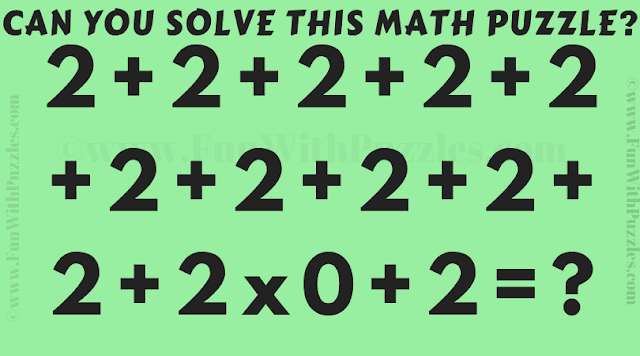This is the Maths Calculations Speed Test Puzzle. This Maths Puzzle, you need to solve as quickly as possible. Note down your time and answer to solve this maths calculation puzzle and then solve it again slowly without any time pressure. See if your answer is different from your previously calculated answer. Also, do not forget to check out our answers. Please post your answer and time to solve this maths speed test puzzle in the comments.Can you solve this speed maths test puzzle?
The answer to this "Maths Calculation Speed Test", can be viewed by clicking on the answer button.

#### 1 comment:

Anonymous said...

The answer is wrong.
The answer should be 2.